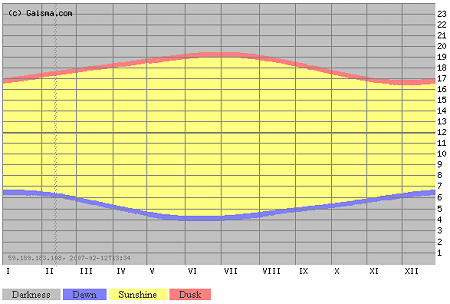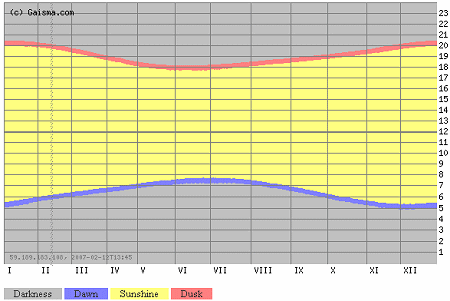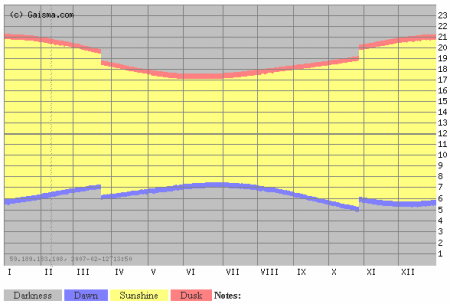Search IntMath
Close

# Gaisma - real-life sine graphs

By Murray Bourne, 13 Feb 2007

Gaisma has

"Sunrise, sunset, dawn and dusk times around the World!".

There is a graph for each city and they are (almost) sine curves.

The yellow portion represents daylight, the gray represents night and the blue and red represent dawn and dusk. The horizontal scale is months in the year and the vertical scale is the hour of the day.

This is the one for Tokyo (northern hemisphere):Capetown, South Africa, is in the southern hemisphere, so the graphs have the opposite shape (in a vertical sense).Many places use daylight saving, and so there is a discontinuity in the graph. For example, Melbourne in Australia has:There are some really strange graphs for places above the arctic (and below the antarctic) circle, like Tasiilaq, Greenland.

Now, the challenge is to model the graphs (find an equation for them), since they aren't exactly sine curves.

Oh, and:

"Gaisma" is a Latvian word, meaning "light".

It's an interesting site.

### Comment Preview

HTML: You can use simple tags like <b>, <a href="...">, etc.

To enter math, you can can either:

1. Use simple calculator-like input in the following format (surround your math in backticks, or qq on tablet or phone):
a^2 = sqrt(b^2 + c^2)
(See more on ASCIIMath syntax); or
2. Use simple LaTeX in the following format. Surround your math with $$ and $$.
$$\int g dx = \sqrt{\frac{a}{b}}$$
(This is standard simple LaTeX.)

NOTE: You can mix both types of math entry in your comment.